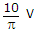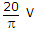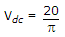# Electronics and Communication Engineering - Analog Electronics - Discussion

5.

A half wave diode circuit using ideal diode has an input voltage 20 sin ωt volts. Then average and rms values of output voltage are

 [A].and 10 V [B].and 10 V [C].and 5 V [D].and 5 V

Explanation:and VC = 0.5 x 20 = 10 V.

 D.Suganya said: (Jun 7, 2014) How to get vdc and rms value?

 Geeta said: (Sep 10, 2014) How to get Vc value?

 Yasobanta said: (Jan 20, 2015) Where to find 0.5?

 Ram said: (Jan 31, 2015) vdc = vav = vm/pi. vrms = vm/2.

 Parth said: (Feb 9, 2015) For Full wave Diode Rectifier Vavg = 2Vm/pi and Vrms = Vm/root(2). For Half wave Diode Vavg = Vm/pi and Vrms = Vm/2.

 Raviraj said: (Mar 17, 2015) Isn't rms value = Vm/sqrt(2)?

 Vinod said: (Jul 3, 2015) The average voltage value of the half wave rectifier = vm/pi.

 Rajan said: (Jun 30, 2016) Actually for AC sine wave V(average) = (2/pi x Vpeak) and V(rms) = Vpeak/sqrt(2), but after the sine wave form past through the Diode with half rectified wave the formula changed slightly with V(dc) = V(average) = Vpeak/pi and V(rms) = 0.45xV(dc). In this example Vpeak = 20, So, Vdc = 20/pi and Vrms = 0.45x (20/pi), that is what I learnt from some ref material.

 Keerthana said: (Jul 13, 2016) Can anyone give a better explanation to understand the solution?

 Dinesh said: (Jan 20, 2017) In between source and load which type component is used to prevent return path in DC source.

 Saivasanth said: (Jun 18, 2017) @Dinesh. Capacitor is used as it blocks dc component.

 Avishkarg said: (Dec 3, 2017) For HWR ckt. avg value=Vm/π and RMS value=Vm/2. For FWR ckt avg value=2Vm/π and RMS value=Vm/√2.

 Sriram said: (Aug 6, 2018) I think the answer should be 40/π.

 Satish M said: (Sep 14, 2018) How to get the answer? Please, anyone, help me.

 Aman Sajwan said: (Jan 10, 2019) Vdc =Vm/π, and Vrms=Vm/2.

 Pen said: (Mar 8, 2019) For HWR; Vrms = 1.57Vavg.

 Chandu Anurag said: (Feb 7, 2020) The average value of half-wave rectifier: vm/pi. The average value of full-wave diode: 2vm /pi.